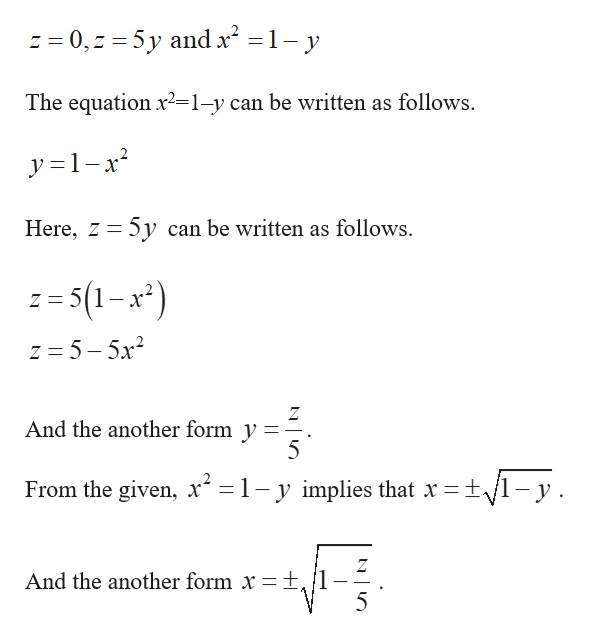# ∭Ef(x,y,z)dV as an iterated integral in six different ways, where E is the solid bounded by z=0,z=5y and x^2=1−y

Question
12 views

∭Ef(x,y,z)dV as an iterated integral in six different ways, where E is the solid bounded by z=0,z=5y and x^2=1−y

check_circle

star
star
star
star
star
1 Rating
Step 1

It is given that the s...help_outlineImage Transcriptionclosez = 0,z 5y and x2 1- y The equation x2=1-y can be written as follows y =1-x Here, z 5y can be written as follows. -5(1-x") Z = z 5-5x2 And the another form y 5 From the given, x = 1- y implies that x =t/1-y Z +, /1 5 And the another form x fullscreen

### Want to see the full answer?

See Solution

#### Want to see this answer and more?

Solutions are written by subject experts who are available 24/7. Questions are typically answered within 1 hour.*

See Solution
*Response times may vary by subject and question.
Tagged in

### Other